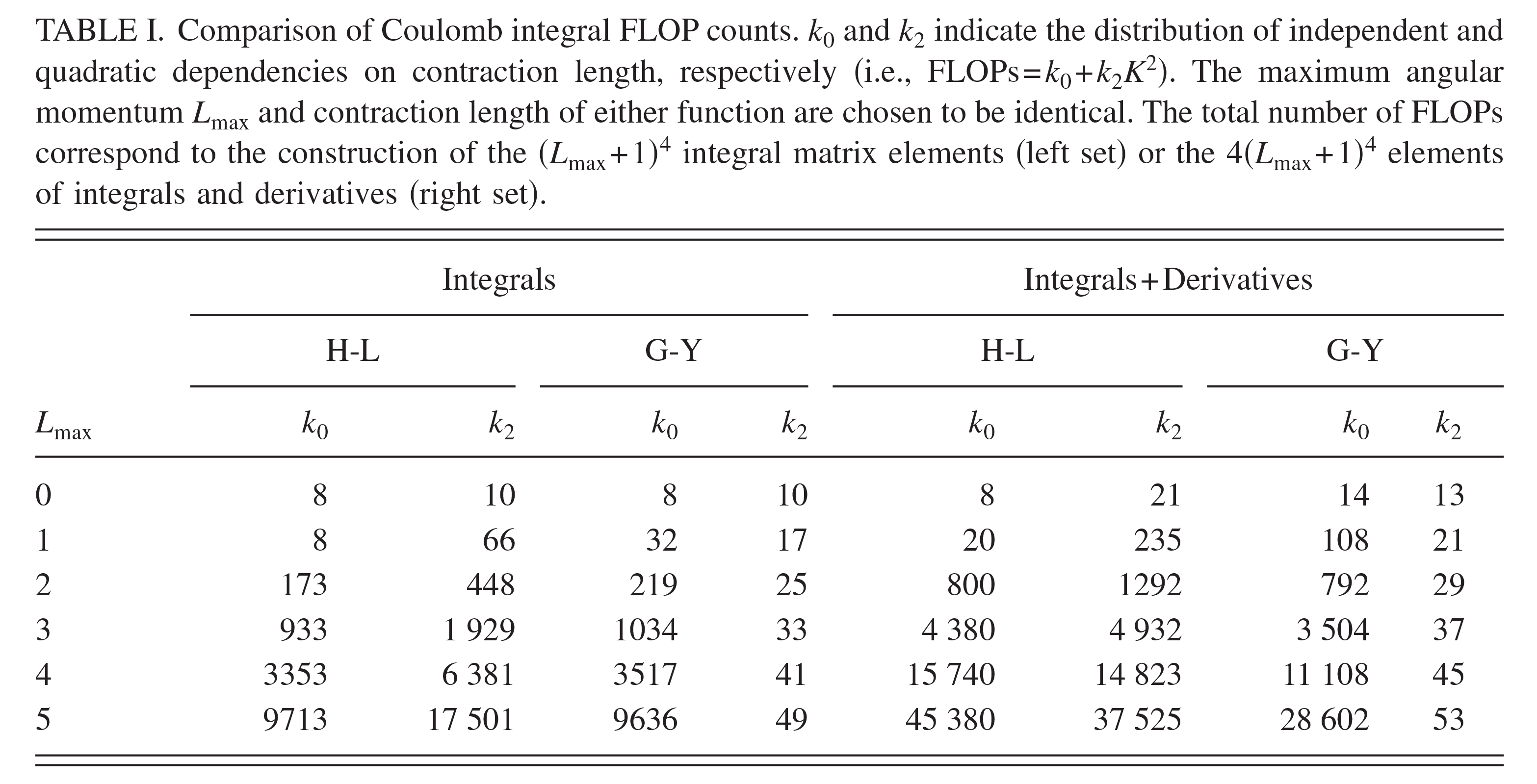## Contracted auxiliary Gaussian basis integral and derivative evaluation

The Journal of Chemical Physics vol. 128  p. 064104  DOI: 10.1063/1.2821745
PMID/PMCID: PMC2735875 Published: 2008-04-14

Timothy J. Giese [ ] , Darrin M. York [ ]### Abstract

The rapid evaluation of two-center Coulomb and overlap integrals between contracted auxiliary solid harmonic Gaussian functions is examined. Integral expressions are derived from the application of Hobson's theorem and Dunlap's product and differentiation rules of the spherical tensor gradient operator. It is shown that inclusion of the primitive normalization constants greatly simplifies the calculation of contracted functions corresponding to a Gaussian multipole expansion of a diffuse charge density. Derivative expressions are presented and it is shown that chain rules are avoided by expressing the derivatives as a linear combination of auxiliary integrals involving no more than five terms. Calculation of integrals and derivatives requires the contraction of a single vector corresponding to the monopolar result and its scalar derivatives. Implementation of the method is discussed and comparison is made with a Cartesian Gaussian-based method. The current method is superior for the evaluation of both integrals and derivatives using either primitive or contracted functions.